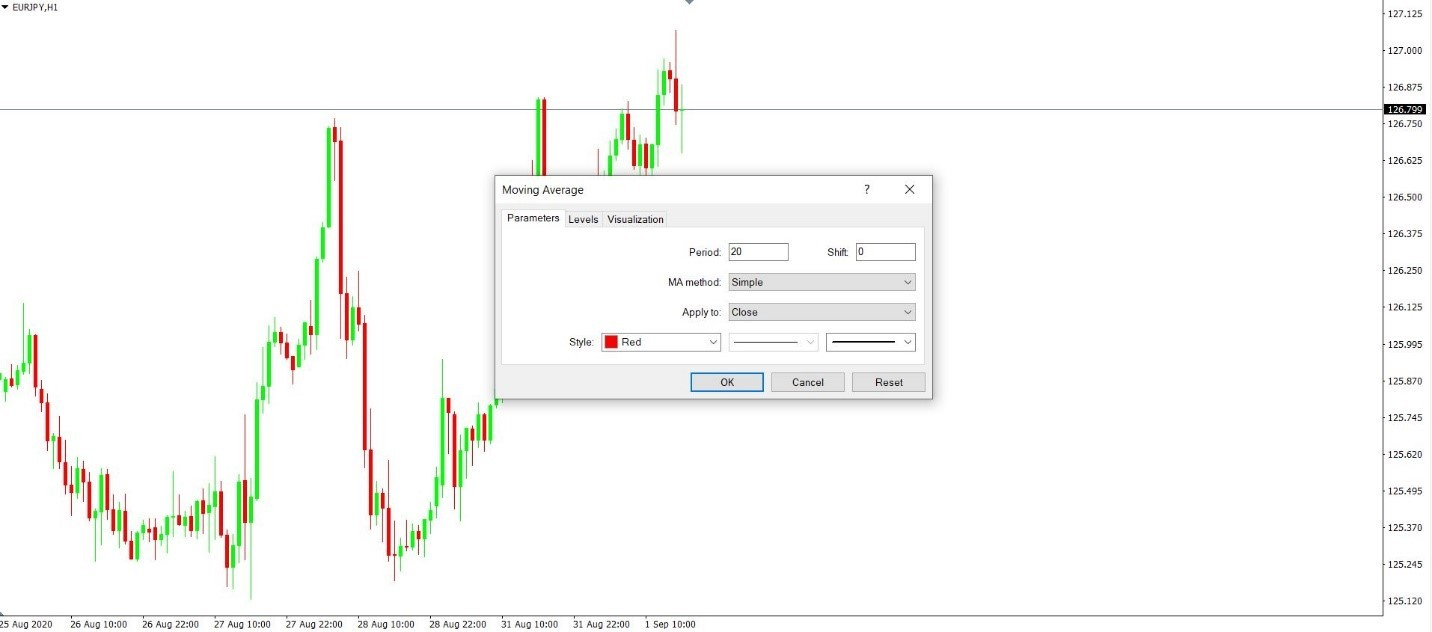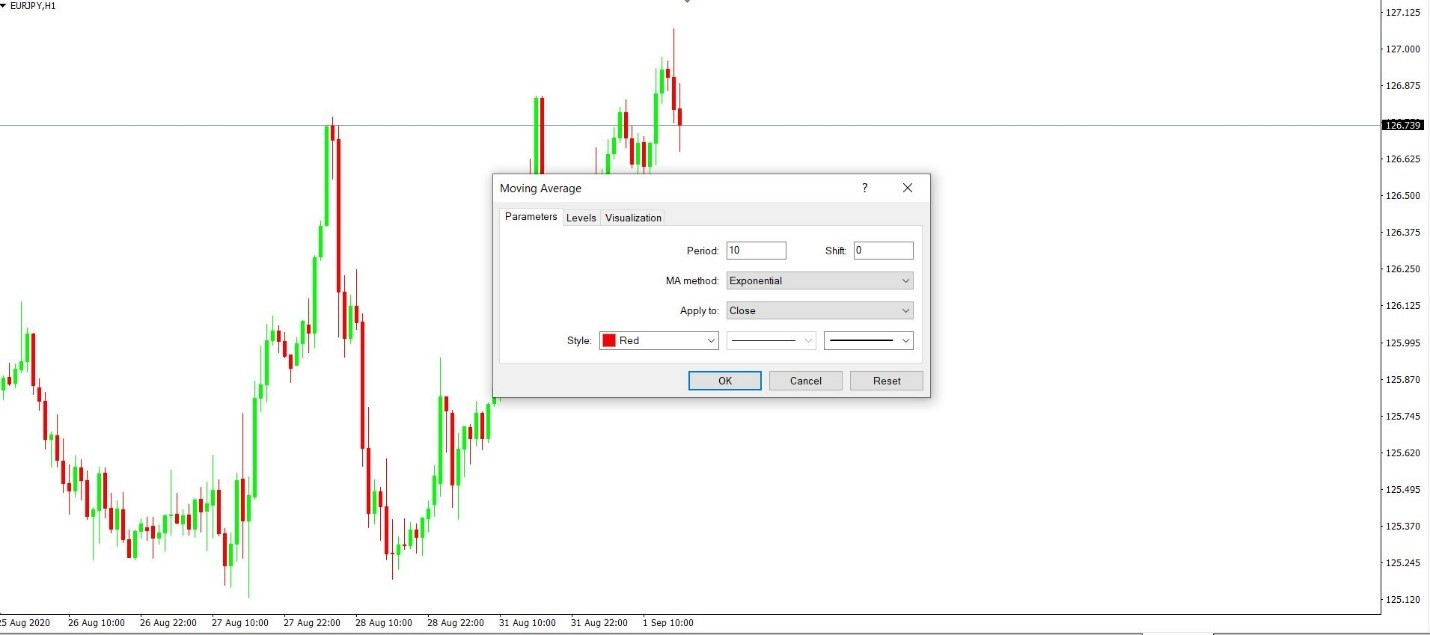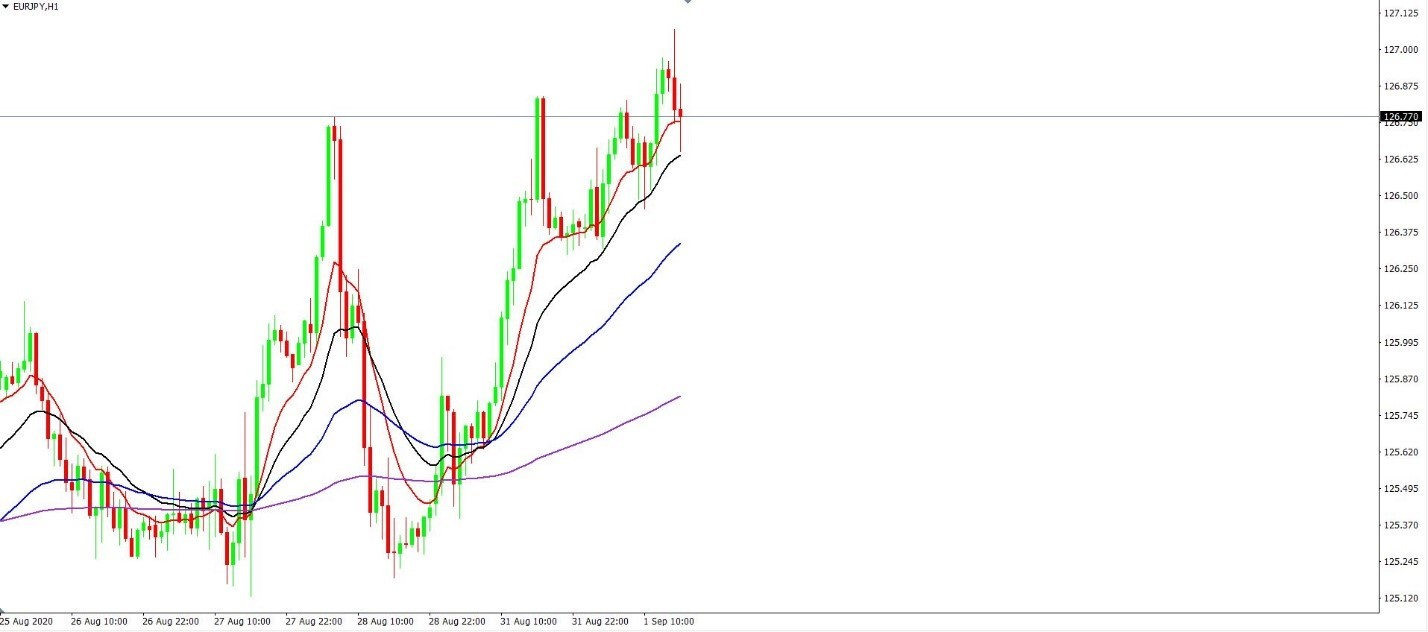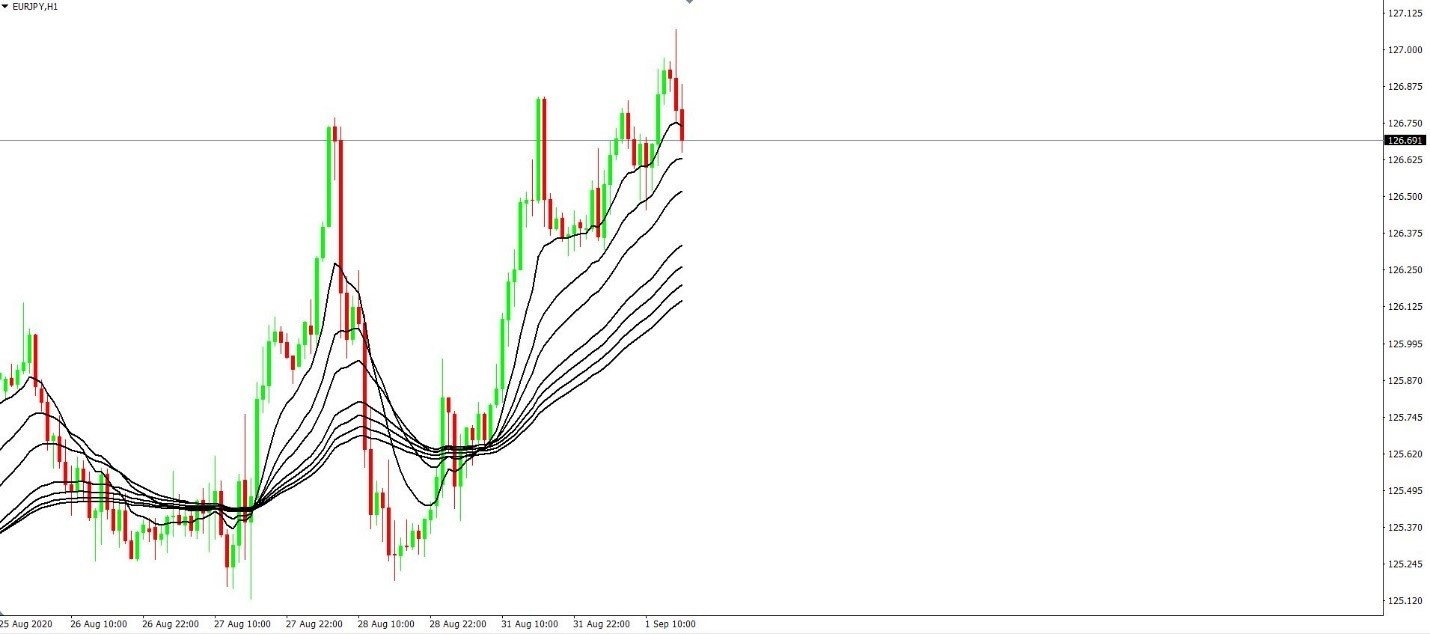What Is an Exponential Moving Average (EMA)?

Share news

Moving averages are trend indicators. They are part of any trading platform and very useful in technical analysis.

Multiple types of moving averages exist. One of the most popular types is the Exponential Moving Average. Also called the EMA, it is preferred by many traders because it reduces the lag or the distance between the price and the average.

One of the most popular trading strategies in the world – EMA

Being trend indicators, moving averages split the trading screen into two different parts – bullish and bearish. The normal interpretation is that the market is bullish while above the moving average, and bearish while below. Also, moving averages act as support and resistance. The bigger the timeframe, the stronger the support or resistance level becomes.

Exponential Moving Average Formula

The exponential moving average formula differs from other moving averages formulas for the simple reason that it puts more weight on the recent price action. In other words, the most recent candlesticks or periods are more important in the EMA formula and they influence the shape of the average.

The EMA formula considers a weighted multiplier calculated as 2 divided by the number of periods considered by the EMA plus 1. For instance, for the EMA(20), the weighted multiplier, k, equals

The formula for EMA

Next, to calculate the EMA(20), or the EMA that considers 20 periods before plotting a value on a chart, use the following formula:

EMA(20)=today’s closing price times the multiplier plus the EMA of the previous period times (1-multiplier).

k = 2/(20 + 1).

Exponential Moving Average Excel Calculation

Consider the table below for the exponential moving average excel calculation:

 EMA(20) Example Today’s closing price t Weighted multiplier k k=2/(20-1) Yesterday’s EMA(20) y Nr. of periods 20 EMA(20) =t*k+y*(1-k)

How to Set Up the Exponential Moving Average In MT4 and MT5 Platforms

The MT4 and MT5 are the most popular trading platforms used by retail traders. Under the Insert/Indicators and Trend, the trend indicators are listed. The Moving Averages tab contains all the moving averages available on the trading platform.

As I mentioned earlier, the EMA is just one of the averages ready to use. A simple moving average or a linear moving average, or even a smoothed moving average exists.

EMA is just one of the averages ready to use in MT4/MT5

Below there is the EURJPY hourly chart. By following the steps I described two paragraphs ago, one can choose to insert the EMA in the chart by simply clicking on the MA method and choosing the Exponential version.Setting Up Different EMA Periods

While still at this point, it is important to remember that one can apply multiple moving averages on a single chart. For instance, if we want to insert the 10 day, 20 day, 50 day, and 200 day EMAs, simply insert in the Parameters box the number of periods and repeat the process four times.

For example, if we change the number of periods with 10, to insert the EMA(10), the Parameters tab looks like below:By clicking OK, the EMA(10) is applied on the chart (with the red color) and by repeating the process you can apply all the other EMAs:As a tip, consider changing the color for the different EMAs so that you will be able to identify them easier. In this case, the EMA(10) is the fastest EMA, with the color red, the next in line is the EMA(20), in black. Next, the EMA(50) follows, in blue, and then the EMA(200), in purple.

As a rule of thumb, traders rarely use moving averages that consider more periods than 200 for the simple reason that the average or the line becomes flat and it loses its relevance.

How I Use the Exponential Moving Average Ribbons Strategy

The ribbons strategy uses a similar principle with the one described above. However, it uses more EMAs – in some cases 8 EMAs and, depending on the type of the investor, the strategy works for scalping, swing trading (position trading) or long-term investing.

A short-term ribbons strategy uses 8 fast EMAs with 10 periods between the EMAs: 10,20,30,40,50,60, 70, 80. The chart below shows the eight EMAs and the idea behind trading with this strategy is that strong trending conditions exist as long as the EMAs remain perfectly aligned. Also, adding to a position on dips into slower EMAs makes sense from the strategy’s point of view.Another strategy I would like to consider is the double exponential moving average strategy. It is similar to the gold and death crosses in classic technical analysis – traders use one fast and one slow EMA and interpret their bullish or bearish crosses.

The Difference Between Simple and Exponential Moving Averages

 SMA – Simple Moving Average EMA – Exponential Moving Average Easy to compute More complex mathematical formula Lagging from the price Reduces the lag considerably More popular More efficient Averages the closing prices Uses most recent price action

Pros of Position Strategy Using the EMA

• Strong support and resistance because of the higher timeframes used
• Ideal for trending markets
• Avoid cross pairs during the Asian session

Cons of Position Strategy Using the EMA

• Takes patience to wait for the price to reach the desired level
• Difficult to interpret when the market is not trending

Key Indicators to Use with the Exponential Moving Average

I think that EMA is one of the most efficient moving average. Because the SMA is more popular, traders tend to forget that the EMA reduces the lag between the candlesticks and the moving averages. Hence, it reaches support and resistance faster and it provides better risk-reward ratios because the distance between the entry price and the invalidation level reduces considerably.

One of the key indicators to use with the exponential moving average is the Bollinger Bands indicator.

This one has three bands – UBB (Upper Bollinger Band), LBB (Lower Bollinger Band), and the MBB (Middle Bollinger Band).

While the UBB and LBB bands cannot be edited, traders can edit the MBB and use an EMA for it. This way, the Bollinger Bands indicator shows more relevant price action when the price reaches the MBB.

My Conclusion

The Exponential Moving Average may be more difficult to compute than other moving averages, but that is not a problem in the 21st century. Trading platforms do the task easily and with a simple mouse click the EMAs are applied on a chart.

The best way to use the EMA indicator is in combination with other oscillators or trend indicators. Also, multiple EMAs on a chart provide a better understanding of what is going on with the price action and when the price effectively breaks support or resistance.

Consider that the more periods the EMA considers, the flatter the moving average becomes. That translates into stronger support and resistance.

Finally, the bigger the timeframe, the bigger the implication for the price reaching the moving average. When that happens on the EMA (200) monthly chart, for example, the chances are that the price will find strong support or resistance.

CFDs are complex instruments and come with a high risk of losing money rapidly due to leverage. CFDs are complex instruments and come with a high risk of losing money rapidly due to leverage. 60% of retail investor accounts lose money when trading CFDs with this provider. You should consider whether you understand how CFDs work, and whether you can afford to take the high risk of losing your money.